Next: The Corrected Asymmetry Up: Measuring the penetration depth with TF-SR Previous: The Role of the Positive Muon

# The Raw Asymmetry For 2-Counter Geometry

Consider the pair of counters i and j in Fig. 3.11, analogous to counters R and L respectively in Fig. 3.10. In a real experiment one can define the raw asymmetry Aij (t) of the appropriately paired histograms Ni (t) and Nj (t) defined by Eq. (3.16) as :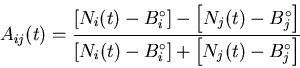(17)

where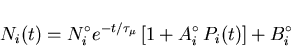(18)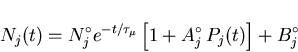(19)

so that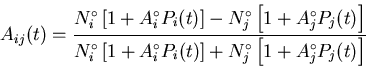(20)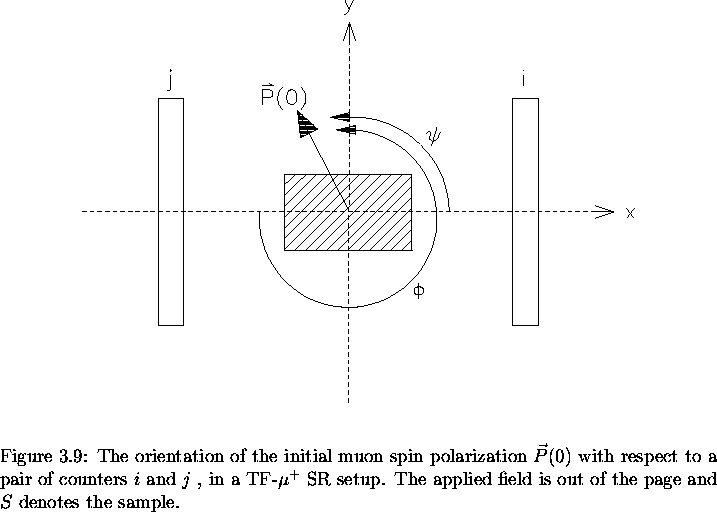The motivation behind introducing Aij (t) is the elimination of the muon lifetime (which is a well known quantity) and the random backgrounds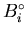and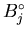. In the ideal situation, the two counters in question are identical to one another so that the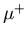SR histograms recorded by each differ only by an initial phase. Because the muons travel for some time in the applied field before reaching the sample, their initial direction of polarization at the time of arrival in the sample is field dependent. However, the difference in phase between histograms is due solely to the geometry of the positron counters with respect to the sample. This situation is illustrated in Fig. 3.11. In the presence of a constant magnetic flux density B, the time evolution of the muon spin polarization vector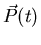is given in general by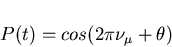(21)

whereis the muon spin precession frequency and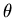is the initial phase of the muon spin polarization vector. For counters i and j in Fig. 3.11, the polarization of the muon spin as seen by each counter is: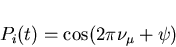(22)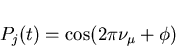(23)

Since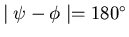then,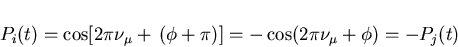(24)

With the counters i and j arranged symmetrically along the x and -x directions: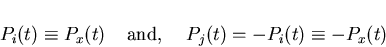(25)

Thus Eq. (3.17) becomes: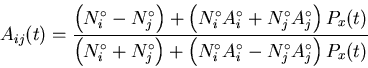(26)

If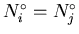and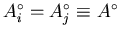then Eq. (3.26) reduces to: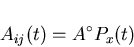(27)

If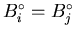, then using Eqs. (3.17), (3.18), (3.19) and (3.27) the x-component of the polarization can be written as: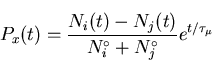(28)Next: The Corrected Asymmetry Up: Measuring the penetration depth with TF-SR Previous: The Role of the Positive Muon
Jess H. Brewer
2001-09-28